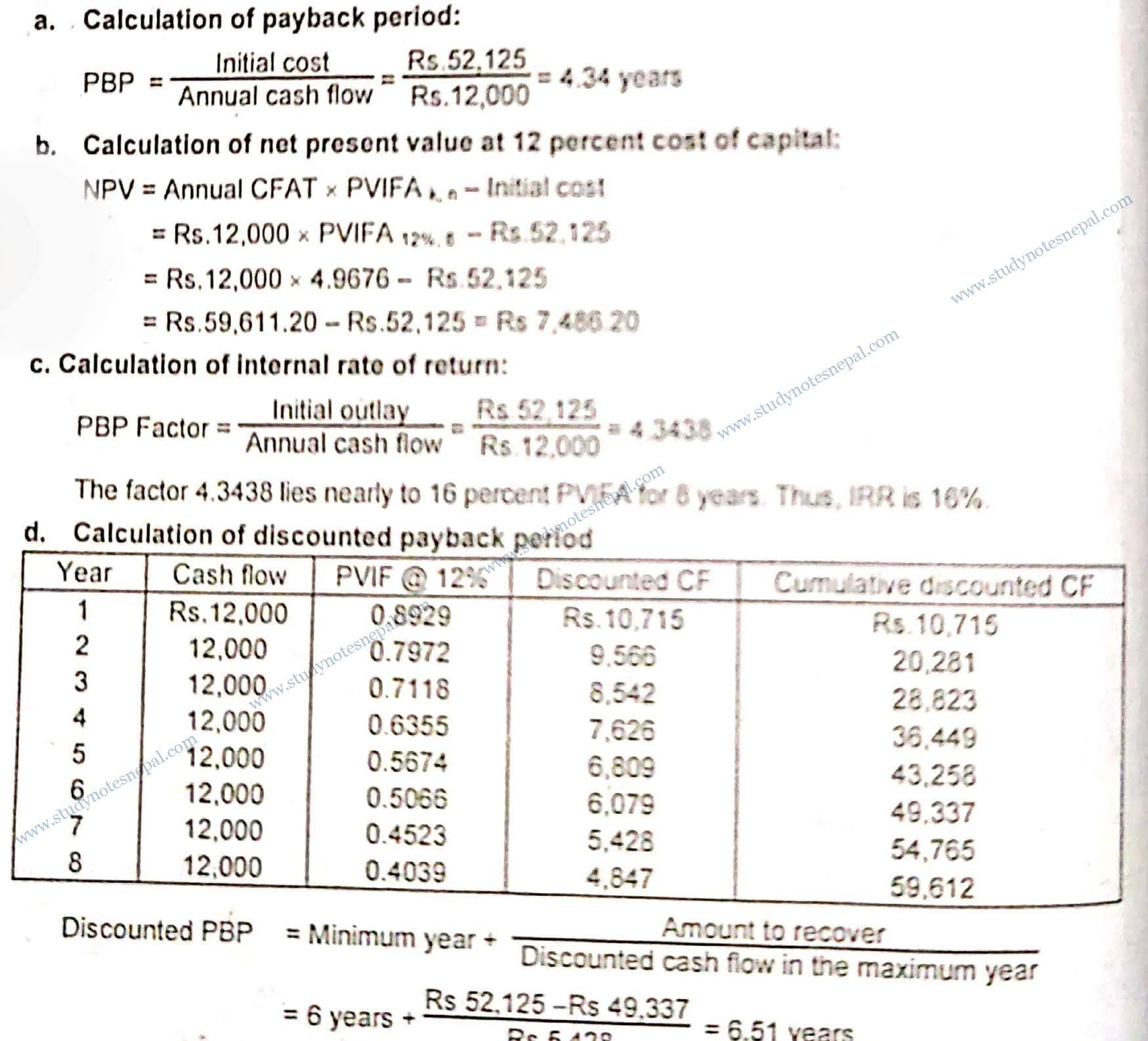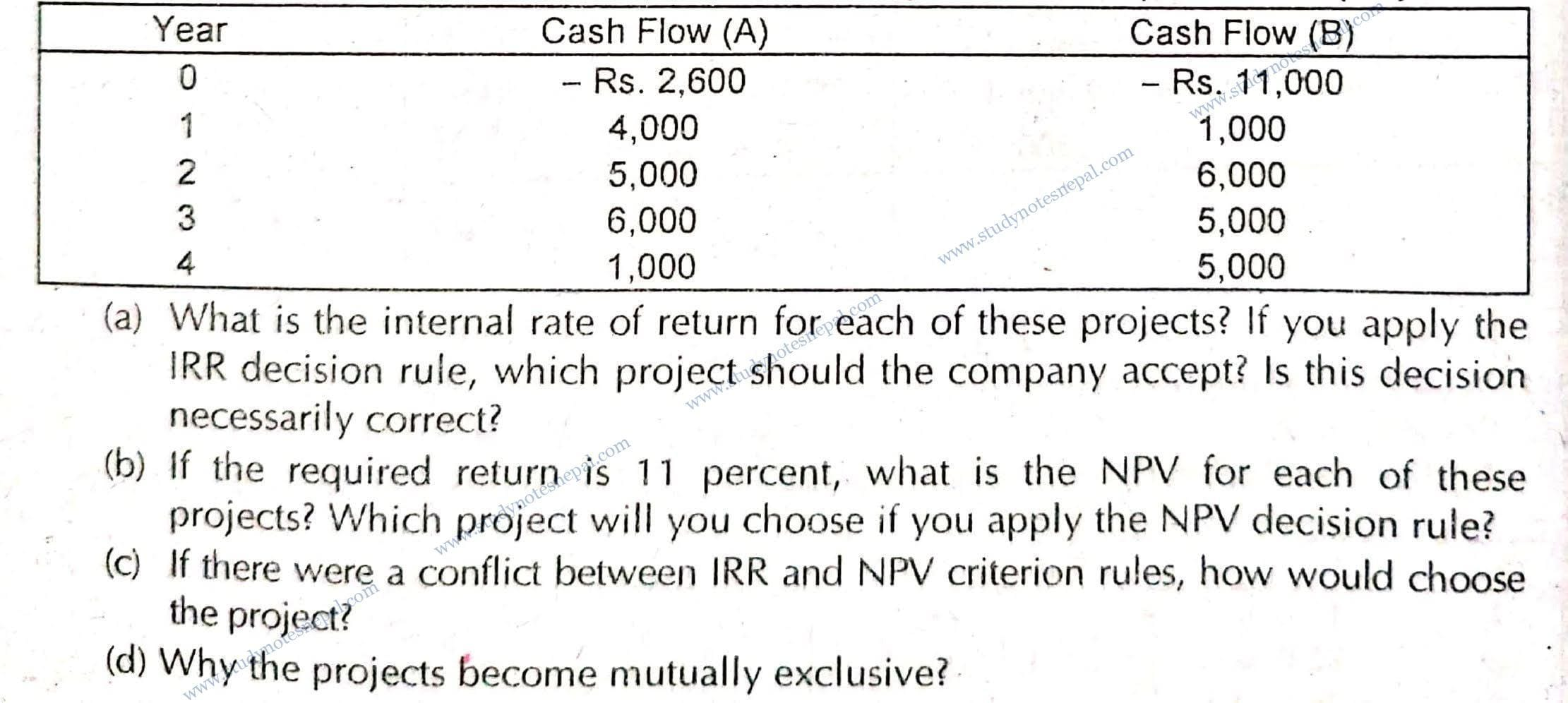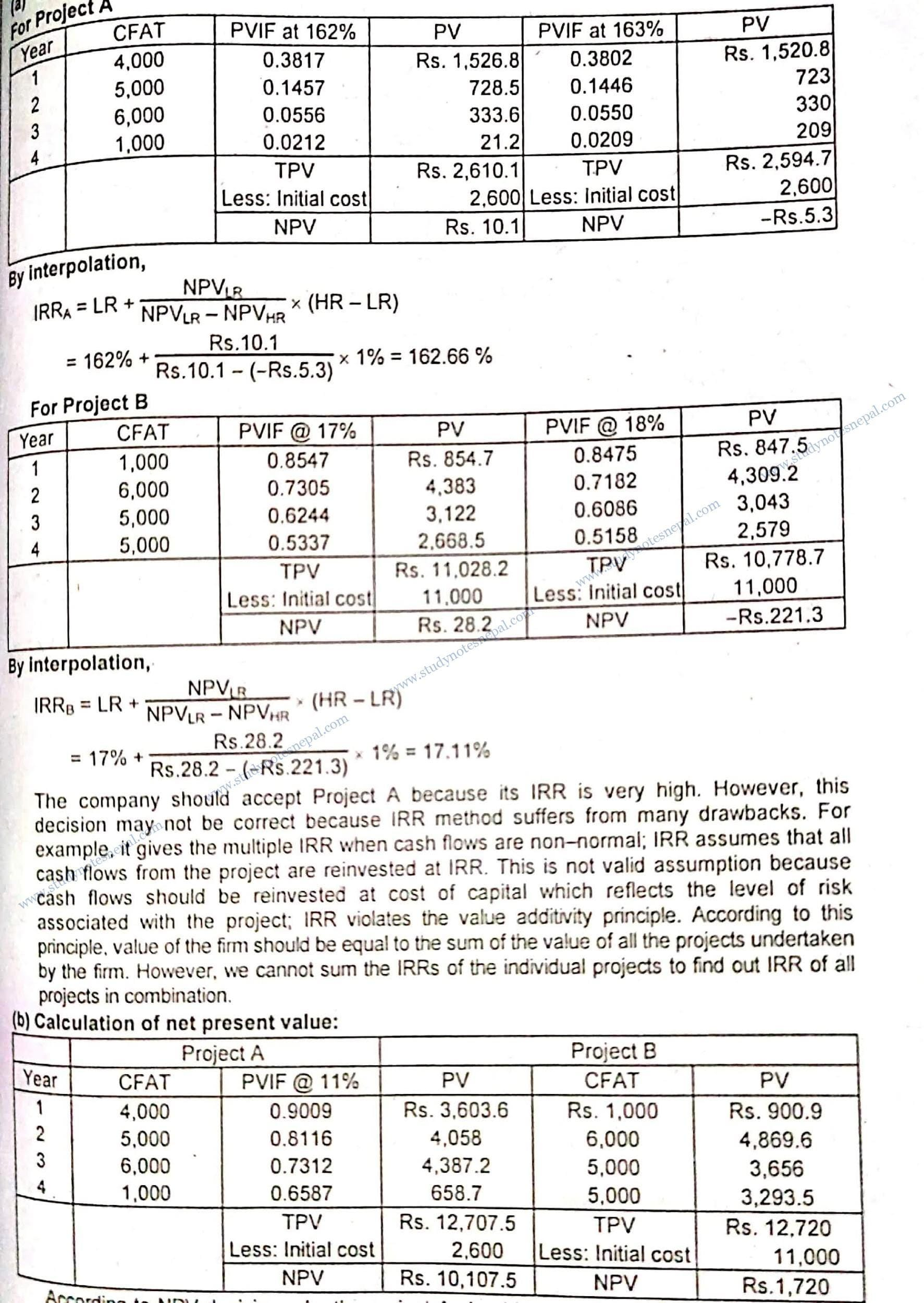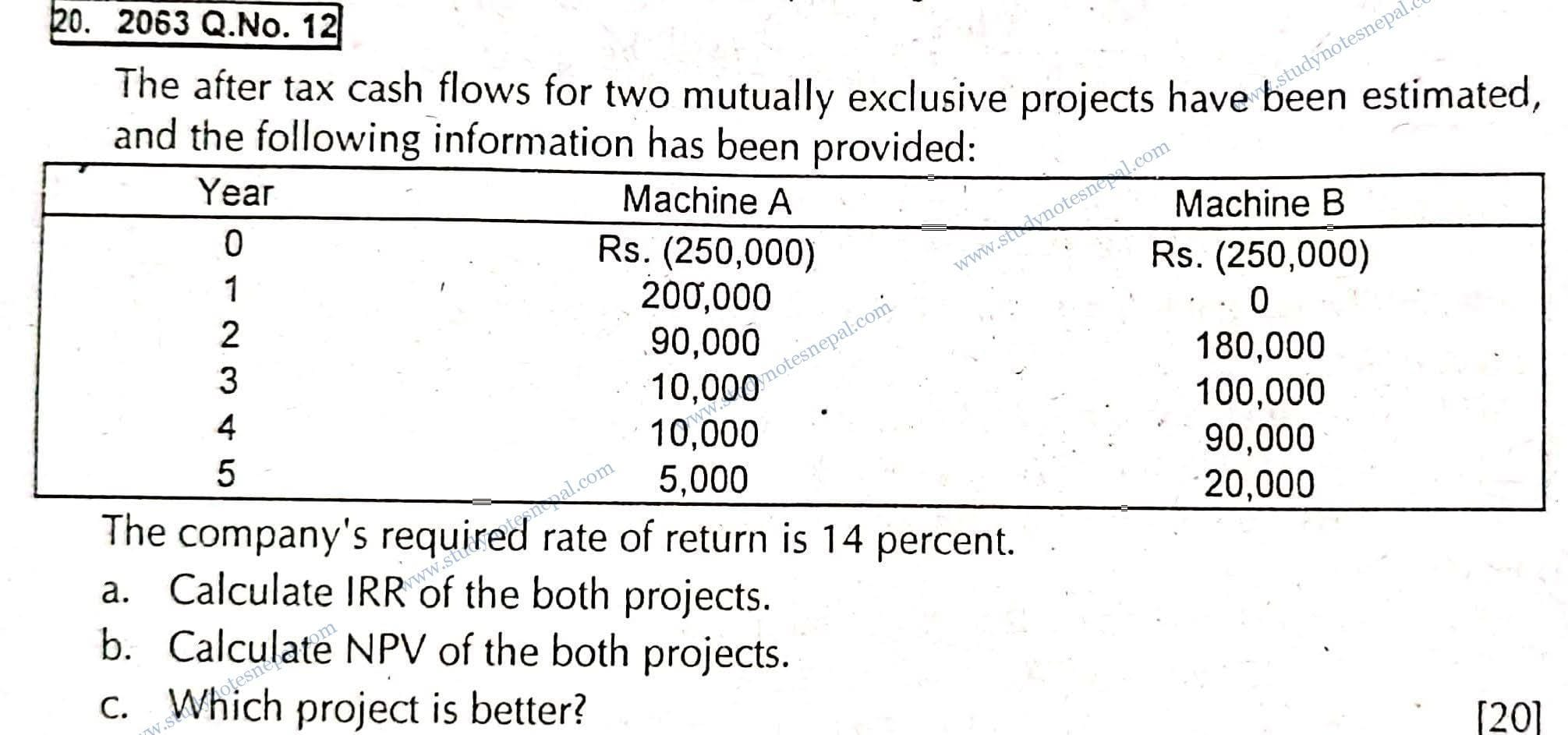###### 1. Briefly explain relative merits and demerits of different methods of evaluating capital budgeting projects.

Capital budgeting projects are evaluated using non-discounted and discounted cash flow methods. Non discounted cash flow method includes the ranking criteria such as payback period and accounting rate of return, whereas the non-discounted cash flow method includes discounted payback, period, net present value and internal rate of return, among the others. The merits and demerits of these methods are described below:

(a) Payback period: The payback period (PBP) is the expected number of years required to recover the initial investment of the project. According to this method, the project with lower PBP is selected. For decision making purposes, the maximum cost recovery time is established, and the payback period of the project is compared with this time. Shorter payback period means earlier recovery of investment. The merits and demerits of PBP method are as follows:

Merits: This method is very simple to understand. In case of the projects, where technological obsolescence is more frequent, investors pay more attention to the quick recovery of investment. Thus, PBP is considered a better project evaluation criterion for such projects.

Demerits: In spite of its simplicity, the PBP has following drawbacks

•  It ignores the cash flows after the recovery of project investment.
• It does not recognize the concept of time value of money.
• It does not consider the level of risk associated with the project.

(b) Discounted payback period: Discounted payback period is the time required to recover the original investment of the project from the discounted cash flow. This technique is a slightly modified version of the regular payback period, where payback period is calculated using discounted cash flows. In other words, this technique I discounts all cash flows at appropriate cost of capital and uses the discounted cash flows to work out the payback period. According to this method also the project with shorter discounted PBP is considered better.

Merits: While payback period does not consider the time value of the money, and risk involved in the project, the discounted PBP considers both.

Demerits: This method also does not consider cash flows beyond the payback year and also does not tell us how much wealth a project adds to the wealth of the shareholders.

(c) Accounting rate of return: Accounting rate of return (ARR), is the profitability measure based on the accounting profit. It is calculated by dividing the average earnings after tax (EAT) by the average investment of the project. According to this method, the ARR is compared against the minimum required rate of return on the project and the project with ARR greater than the minimum required rate of return is accepted.

Merits: This method is also simple to understand and easy to calculate. Since this method is based on the accounting information, the required accounting information is easily available.

Demerits: Some of the drawbacks of ARR are as follows:

• ARR is just a ratio between two accounting figures rather than the true rate of return.
• It also ignores the concept of time value of money.
• There is no standardized method to fix the minimum required rate of return to compare against ARR.
• This method is based on accounting profits rather than cash flows so it does represent the true profitability in an economic sense.

(d) Net present value: Net present value (NPV) is one of the widely used discounted cash flow techniques of evaluating capital budgeting projects. NPV of the project is the difference between present value of cash inflows and outflows. According to this method, benefits of the project measured in terms of cash flow are discounted, and summed up, and then initial cash outlay of the project is deducted. The remaining value is known as net present value. The project with positive NPV is accepted under this method. A positive NPV means positive contribution to the value of the firm and wealth of the shareholders.

Merits: NPV overcomes the drawbacks of all techniques described earlier. The merits of NPV method are as follows:

• It recognizes the concept of time value of money.
• It takes into account all cash flows over the life of the project.
• It considers the level of risk associated with the project as selection of the appropriate discount rate based on the project risk.
•  This method is based on the cash flows rather than accounting profits.
• It is consistent with the shareholders’ wealth maximization objective.

Demerits: However, NPV also suffers from following disadvantages:

• This method is based on the expected cash flow of the project. However, in real life it is very difficult to forecast the cash flows with accuracy.
• NPV is sensitive to discount rate and the selection of the appropriate discount rate is a difficult task under this method.
• It does not give appropriate ranking of the projects with unequal lives and unequal size of investments.

(e) Internal rate of return: Internal rate of return (IRR) is the discount rate that makes the present value of cash inflows equal to the present value of the cash outflows of the project. In other words, IRR is the discount rate producing zero NPV of the projects. This method is also based on the discounted cash flows and equally likely to the NPV method. According to this method, a project is accepted if its IRR is greater than the cost of capital.

Merits: Some merits of IRR methods are as follows:

• This method is based on the cash flows rather than accounting profits.
• It also recognizes the concept of time value of money.
• It also takes into account all cash flows over the life of the project.
• It considers the level of risk associated with a project as IRR is compared against cost of capital.
• It is also consistent with the shareholders’ wealth maximization objective.
• IRR is easy to understand and communicate to the management because it is expressed in percent rather than in terms of money.

Demerits: Some of the shortcomings of IRR method are as follows:

• IRR method gives the multiple IRR when cash flows are non-normal.
•  IRR assumes that all cash flows from the project are reinvested at IRR. This is not a valid assumption because cash flows should be reinvested at cost of capital which reflects the level of risk associated with the project.
• IRR violates the value additivity principle. According to this principle, the value of the firm should be equal to the sum of the value of all the projects undertaken by the firm. However, we cannot sum the IRRs of the individual projects to find out the IRR of all projects in combination.
• To sum up, the NPV method does not have all these shortcomings of IRR. In other words, the NPV method holds the value additivity principle, and assumes an appropriate reinvestment rate. So, the NPV method is considered superior.

#### Write notes on

###### a) Net present value

Net present value (NPV) is one of the widely used discounted cash flow techniques of evaluating capital budgeting projects. NPV is the difference between present value of cash inflows and outflows from the project. According to this method, benefits of the project measured in terms of cash flow are discounted, and sum up, and then initial cash outlay of the project is deducted. The remaining value is known as net present value. The project with positive NPV is accepted under this method. A positive NPV means positive contribution to the value of the firm and wealth of the shareholders.

Merits: NPV overcomes the drawbacks of all other techniques of evaluating capital projects. The merits of NPV method are as follows:

• It recognizes the concept of time value of money.
•  It takes into account all cash flows over the life of the project.
• It considers the level of risk associated with the project as selection of the appropriate discount rate based on the project risk.
• This method is based on the cash flows rather than accounting profits.
• It is consistent with the shareholders’ wealth maximization objective

Demerits: However, NPV also suffers from following disadvantages:

• This method is based on the expected cash flow of the project. However, in real life it is very difficult to forecast the cash flows with accuracy.
• NPV is sensitive to discount rate and the selection of the appropriate discount
• rate is a difficult task under this method.
• It does not give appropriate ranking of the projects with unequal lives and unequal size of investments.
###### b) Crossover rate

Crossover rate is the discount rate at which two mutually exclusive projects have equal net present value. In case of two mutually exclusive projects, the NPV of one project may be higher than that of the other at a lower cost of capital, while the NPV of the same project may be lower than that of another at a higher cost of capital. In this situation, there exists a crossover rate at which NPVs of two projects remain equal. If the NPV of one project remains always higher than that of another at any level of cost of capital then there exists no crossover rate.

In  the existence of crossover rate, NPV and IRR methods give conflicting ranking of the mutually exclusive projects. It happens when the cost of capital is less than the crossover rate. In contrast, if cost of capital is greater than the crossover rate, there exists no conflict in ranking by NPV and IRR method. As we know that NPV is sensitive to the change in cost of capital whereas IRR is not. NPV of some projects may be more sensitive to cost of capital than the other. These factors combined together cause the existence of crossover rate.

###### c) Classification of capital projects

Capital projects are classified in a number of ways. Some of them are discussed below:

(i) Independent projects: Two projects are said to be independent if selection of one project does not prevent the selection of another project. Such projects can be evaluated independently because their cash flows are independent on each other. For example, establishment of a Bakery Industry and establishment of Automobile Assembling Industry are independent projects.

(ii) Dependent projects: Two projects are said to be dependent if cash flows of one project are affected by another. In case of dependent projects, acceptance of one forces the acceptance of another. For example, establishing an automobile showroom and establishing an automobile service center are dependent projects.

(iii) Mutually exclusive projects: Two projects are said to be mutually exclusive if acceptance of one excludes the other. In case of mutually exclusive projects only the best one is selected. For example, buying an IBM photocopy machine versus a Samsung photocopy machine represents mutually exclusive projects, because we buy the best one among these two.

(iv) Replacement project: A replacement project is one in which existing assets are replaced with new one. When existing assets turned out to be less efficient due to wear and tear and due to the advent of new technology they are replaced with newer, much more efficient one. Replacement projects are considered to maintain the efficiency of operation and to reduce the cost.

(v) Expansion project: The project that is undertaken to expand the operation of business is called expansion project. The expansion may take place in production and distribution capacity if the current capacity is insufficient to meet growing market demand.

1) Project L has a cost of Rs 52,125, and its expected net cash inflows are Rs 12,000 per year for 8 years.

a) What is the project’s payback period?

b) The cost of capital is 12 percent. What is the project’s net present value?

c) What is the project’s internal rate of return?

d)What is the project’s discounted payback period, assuming a 12 percent cost of capital?2) Bagmati Engineering is considering the purchase of two pieces of equipment, X and Y, in this year’s capital budget. The projects are not mutually exclusive. The cash outlay for the X is Rs 17,100, and that for the Y is Rs 22,430. The firm’s cost of capital is 14 percent. After tax cash flows, including depreciation, are as follows:

Since the equipment is not mutually exclusive, the firm can accept both of them provided. that there is no capital constraint, because both the equipment have positive NPVs and IRRS greater than cost of capital.

3) Bheri Chemical Company is considering the replacement of two old machines with a new, more efficient machine. It has determined that the relevant after-tax incremental operating cash flows of this replacement proposal are as follows:4) Generation X, Inc., has identified the following two mutually exclusive projects:SOLUTION

(a) Calculation of internal rate of return (IRR):

For Project AAccording to the NPV decision rule, project A should be accepted because it has a higher positive net present value.

(c) If there were a conflict between the NPV and IRR. The decision according to the NPV method should be recommended because NPV is superior to the IRR method.

(d) If two projects substitute each other or serve the same purpose, they become mutually exclusive. In the case of mutually exclusive projects we select the best one.

5) The after tax cash flows for two mutually exclusive projects have been estimated, and the following information has been provided:###### 1. Briefly explain relative merits and demerits of different methods of evaluating capital budgeting projects.

Capital budgeting projects are evaluated using non-discounted and discounted cash flow methods. Non discounted cash flow method includes the ranking criteria such as payback period and accounting rate of return, whereas non-discounted cash flow method includes discounted payback, period, net present value and internal rate of return, among the others. The merits and demerits of these methods are described below:

(a) Payback period: The payback period (PBP) is the expected number of years required to recover the initial investment of the project. According to this method, the project with lower PBP is selected. For decision making purposes, the maximum cost recovery time is established, and payback period of the project is compared with this time. Shorter payback period means earlier recovery of investment. The merits and demerits of PBP method are as follows:

Merits: This method is very simple to understand. In case of the projects, where technological obsolescence is more frequent, investors pay more attention to the quick recovery of investment. Thus, PBP is considered a better project evaluation criterion for such projects.

Demerits: In spite of its simplicity, the PBP has following drawbacks

•  It ignores the cash flows after the recovery of project investment.
• It does not recognize the concept of time value of money.
• It does not consider the level of risk associated with the project.

(b) Discounted payback period: Discounted payback period is the time required to recover the original investment of the project from the discounted cash flow. This technique is a slightly modified version of regular payback period, where payback period is calculated using discounted cash flows. In other words, this technique I discounts all cash flows at appropriate cost of capital and uses the discounted cash flows to work out the payback period. According to this method also the project with shorter discounted PBP is considered better.

Merits: While payback period does not consider the time value of the money, and risk involved in the project, the discounted PBP considers both.

Demerits: This method also does not consider cash flows beyond the payback year and also does not tell us how much wealth a project adds to the wealth of the shareholders.

(c) Accounting rate of return: Accounting rate of return (ARR), is the profitability measure based on the accounting profit. It is calculated by dividing the average earnings after tax (EAT) by the average investment of the project. According to this method, the ARR is compared against the minimum required rate of return on the project and the project with ARR greater than the minimum required rate of return is accepted.

Merits: This method is also simple to understand and easy to calculate. Since this method is based on the accounting information, the required accounting information is easily available.

Demerits: Some of the drawbacks of ARR are as follows:

• ARR is just a ratio between two accounting figures rather than the true rate of return.
• It also ignores the concept of time value of money.
• There is no standardized method to fix the minimum required rate of return to compare against ARR.
• This method is based on accounting profits rather than cash flows so it does represent the true profitability in an economic sense.

(d) Net present value: Net present value (NPV) is one of the widely used discounted cash flow techniques of evaluating capital budgeting projects. NPV of the project is the difference between present value of cash inflows and outflows. According to this method, benefits of the project measured in terms of cash flow are discounted, and sum up, and then initial cash outlay of the project is deducted. The remaining value is known as net present value. The project with positive NPV is accepted under this method. A positive NPV means positive contribution to the value of the firm and wealth of the shareholders.

Merits: NPV overcomes the drawbacks of all techniques described earlier. The merits of NPV method are as follows:

• It recognizes the concept of time value of money.
• It takes into account all cash flows over the life of the project.
• It considers the level of risk associated with the project as selection of the appropriate discount rate based on the project risk.
•  This method is based on the cash flows rather than accounting profits.
• It is consistent with the shareholders’ wealth maximization objective.

Demerits: However, NPV also suffers from following disadvantages:

• This method is based on the expected cash flow of the project. However, in real life it is very difficult to forecast the cash flows with accuracy.
• NPV is sensitive to discount rate and the selection of the appropriate discount rate is a difficult task under this method.
• It does not give appropriate ranking of the projects with unequal lives and unequal size of investments.

(e) Internal rate of return: Internal rate of return (IRR) is the discount rate that makes the present value of cash inflows equal to the present value of the cash outflows of the project. In other words, IRR is the discount rate producing zero NPV of the projects. This method is also based on the discounted cash flows and equally likely to the NPV method. According to this method, a project is accepted if its IRR is greater than the cost of capital.

Merits: Some merits of IRR methods are as follows:

• This method is based on the cash flows rather than accounting profits.
• It also recognizes the concept of time value of money.
• It also takes into account all cash flows over the life of the project.
• It considers the level of risk associated with a project as IRR is compared against cost of capital.
• It is also consistent with the shareholders’ wealth maximization objective.
• IRR is easy to understand and communicate to the management because it is expressed in percent rather than in terms of money.

Demerits: Some of the shortcomings of IRR method are as follows:

• IRR method gives the multiple IRR when cash flows are non-normal.
•  IRR assumes that all cash flows from the project are reinvested at IRR. This is not a valid assumption because cash flows should be reinvested at cost of capital which reflects the level of risk associated with the project.
• IRR violates the value additivity principle. According to this principle, the value of the firm should be equal to the sum of the value of all the projects undertaken by the firm. However, we cannot sum the IRRs of the individual projects to find out the IRR of all projects in combination.
• To sum up, the NPV method does not have all these shortcomings of IRR. In other words, the NPV method holds the value additivity principle, and assumes an appropriate reinvestment rate. So, the NPV method is considered superior.